# 三个优秀的PyTorch实现语义分割框架

09/01 11:00

1. 下载VOC数据集，将 JPEGImages SegmentationClass两个文件夹放入到data文件夹下。
2. 终端切换到目标目录，运行 python train.py -h查看训练
(torch) qust116-jq@qustx-X299-WU8:~/语义分割\$ python train.py -husage: train.py [-h] [-m {Unet,FCN,Deeplab}] [-g GPU]choose the modeloptional arguments:  -h, --help            show this help message and exit  -m {Unet,FCN,Deeplab}, --model {Unet,FCN,Deeplab}                        输入模型名字  -g GPU, --gpu GPU     输入所需GPU

1. 预测需要手动修改 predict.py中的模型

## 2 数据集

VOC数据集一般是用来做目标检测，在2012版本中，加入了语义分割任务。

PASCAL VOC分割任务中，共有20个类别的对象，其他内容作为背景类，其中红色代表飞机类，黑色是背景，飞机边界部分用米黄色（看着像白色）线条描绘，表示分割模糊区。

%matplotlib inlineimport torchimport torchvisionfrom torch import nnfrom torch.nn import functional as Ffrom d2l import torch as d2l

### 3.1 网络结构

pretrained_net = torchvision.models.resnet18(pretrained=True)list(pretrained_net.children())[-3:]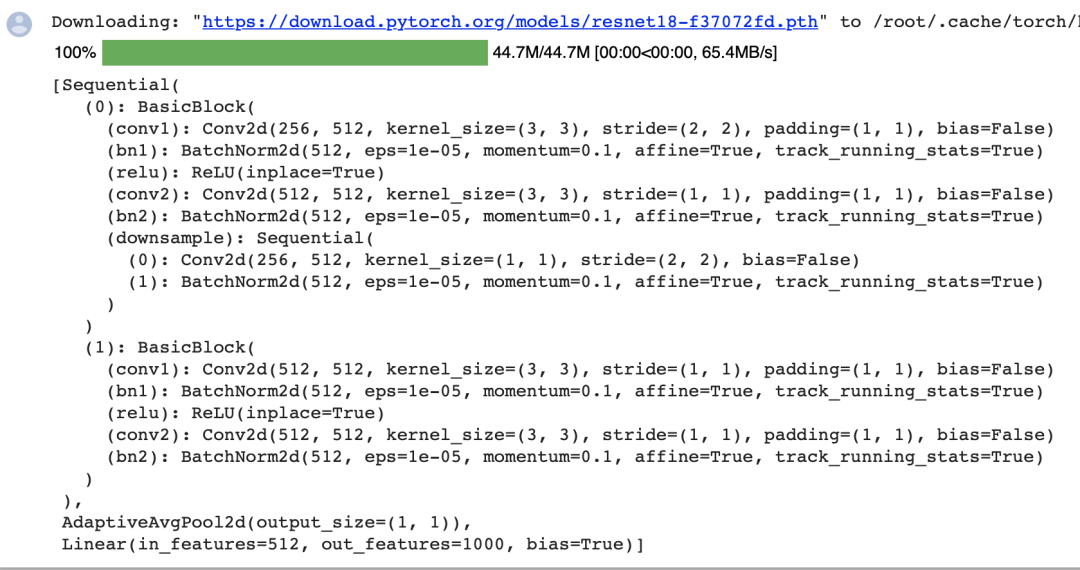创建一个全卷积网络实例net。它复制了Resnet-18中大部分的预训练层，但除去最终的全局平均汇聚层和最接近输出的全连接层。

net = nn.Sequential(*list(pretrained_net.children())[:-2])

X = torch.rand(size=(1, 3, 320, 480))net(X).shape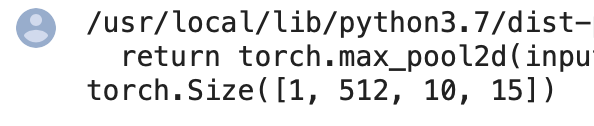使用 卷积层将输出通道数转换为Pascal VOC2012数据集的类数（21类)。最后，我们需要将要素地图的高度和宽度增加32倍，从而将其变回输入图像的高和宽。

num_classes = 21net.add_module('final_conv', nn.Conv2d(512, num_classes, kernel_size=1))net.add_module('transpose_conv', nn.ConvTranspose2d(num_classes, num_classes,                                    kernel_size=64, padding=16, stride=32))

### 3.2 初始化转置卷积层

def bilinear_kernel(in_channels, out_channels, kernel_size):    factor = (kernel_size + 1) // 2    if kernel_size % 2 == 1:        center = factor - 1    else:        center = factor - 0.5    og = (torch.arange(kernel_size).reshape(-1, 1),          torch.arange(kernel_size).reshape(1, -1))    filt = (1 - torch.abs(og - center) / factor) * \           (1 - torch.abs(og - center) / factor)    weight = torch.zeros((in_channels, out_channels,                          kernel_size, kernel_size))    weight[range(in_channels), range(out_channels), :, :] = filt    return weight

conv_trans = nn.ConvTranspose2d(3, 3, kernel_size=4, padding=1, stride=2,                                bias=False)conv_trans.weight.data.copy_(bilinear_kernel(3, 3, 4));

W = bilinear_kernel(num_classes, num_classes, 64)net.transpose_conv.weight.data.copy_(W);

### 3.3 训练

def loss(inputs, targets):    return F.cross_entropy(inputs, targets, reduction='none').mean(1).mean(1)num_epochs, lr, wd, devices = 5, 0.001, 1e-3, d2l.try_all_gpus()trainer = torch.optim.SGD(net.parameters(), lr=lr, weight_decay=wd)d2l.train_ch13(net, train_iter, test_iter, loss, trainer, num_epochs, devices)

## 4 开源代码和Dataset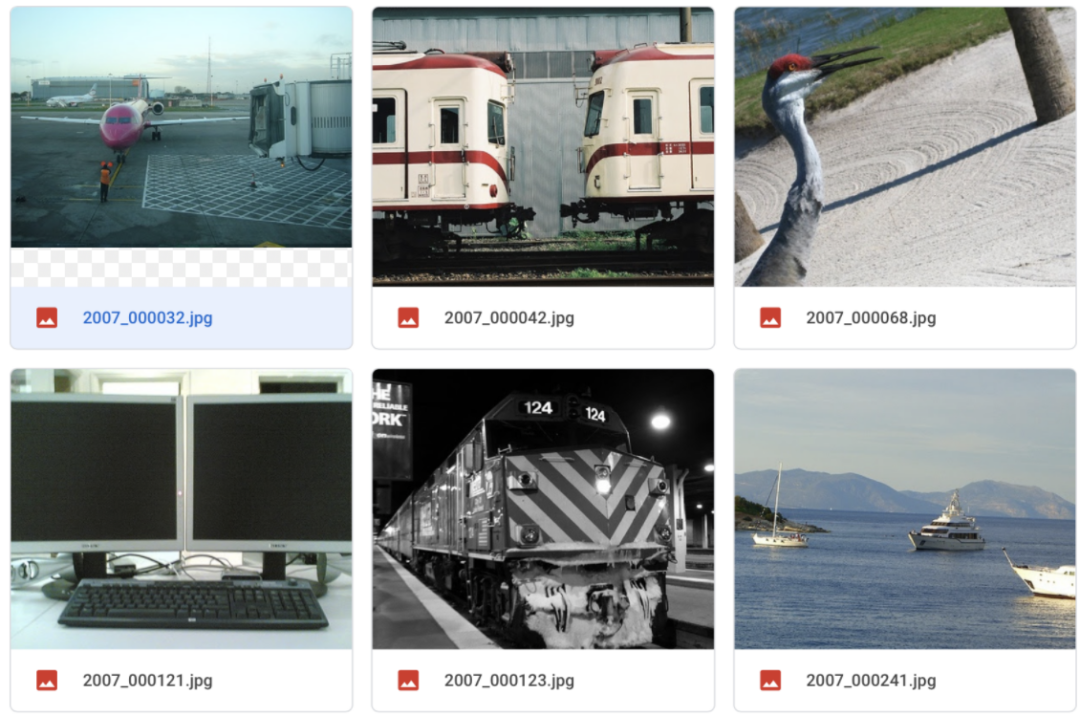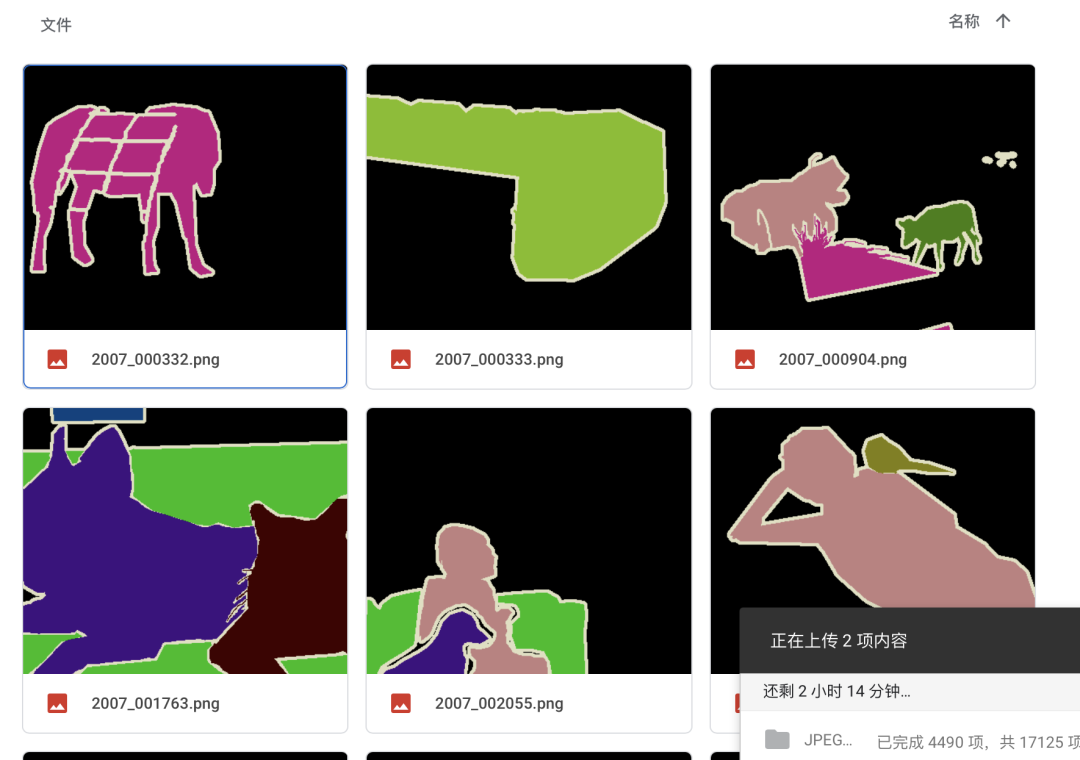!python3 train.py -m Unet -g 0

DeeplabV3分割结果：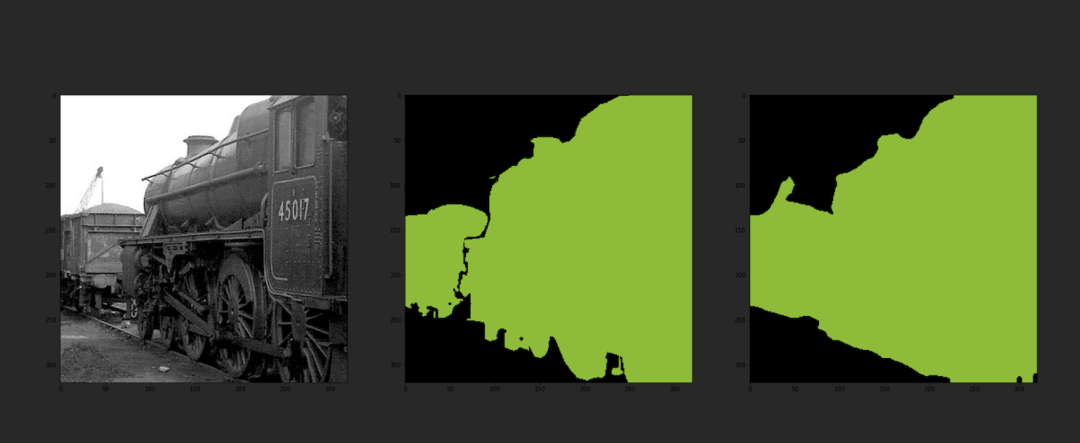FCN分割结果：

U-Net分割结果：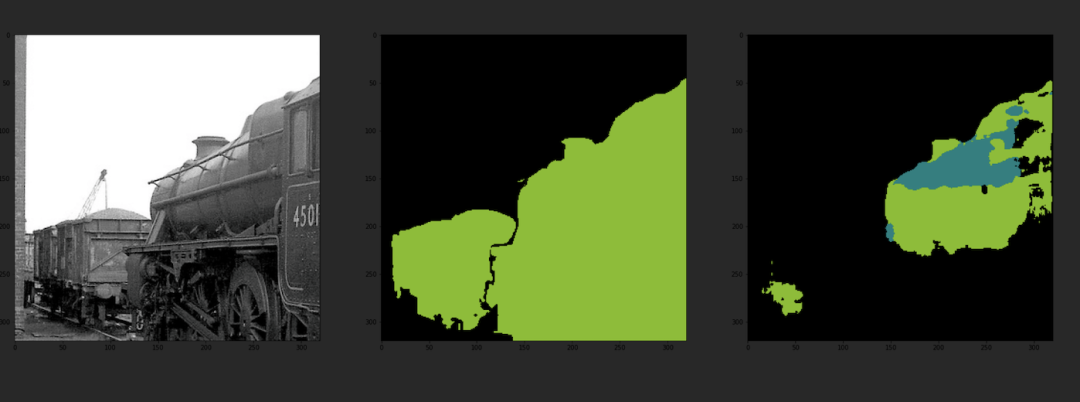## 6 参考

.https://zh-v2.d2l.ai/index.html

搜索公众号添加： datanlpTensorFlow 2.0深度学习案例实战

《基于深度学习的自然语言处理》中/英PDF

Deep Learning 中文版初版-周志华团队

【全套视频课】最全的目标检测算法系列讲解，通俗易懂！

《美团机器学习实践》_美团算法团队.pdf

《深度学习入门：基于Python的理论与实现》高清中文PDF+源码

2019最新《PyTorch自然语言处理》英、中文版PDF+源码

yolo3 检测出图像中的不规则汉字

VGG16迁移学习，实现医学图像识别分类工程项目

Machine Learning Yearning 中文翻译稿

搜索公众号添加： datayx0
0 收藏

### 作者的其它热门文章0 评论
0 收藏
0